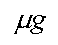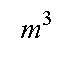Module 1, Sub-module 1E, Page 1

Dose, Chemical units.

Here is a review of some common chemical units as they are applied to toxicology.

Dose per Body Weight

Tests are done on batches of animals of the same strain and species and similar age and weight. But, animals within a batch often differ in body weight. When toxicologists poison lab animals, we frequently express the dose as mg/kg. That means milligrams of the poison per kilogram of animal's body weight.

So if we are testing at 100 mg/ kg, how much do I feed a 300 gm rat Click here if you want to see that calculated. Use your browser's BACK button to return here. The measure here is mass of chemical per mass of animal.

Water Solutions

Often we want to express the amount of something in solution. For example, the maximum contaminant level of arsenic in drinking water is 50 micrograms per liter. The symbol for microgram is the lower case Greek mu followed by g like thisbut most browsers do not support the mu. You will sometimes see a lower case "u" used for this, ug, which is what I will have to do. Now we have 50 ug of arsenic per liter, but what is the substance in the liter? You are tempted to say "water." But when we are discussing concentrations, we mean liter of solution. If we wanted to make a test solution of 50 ug arsenic per liter, we start by weighing 50 ug of arsenic into a dry beaker (volumetric flask would be better), then adding water until the solution is exactly one liter. You recognize the the true volume of water is slightly less than one liter. The measure here is mass of contaminant per volume.

Solid mixtures

Often risks are related to contaminated soil - dirty dirt. We call the soil the "matrix" and the chemical (or chemicals) the contaminant. Here we start by weighing the combination, matrix plus contaminant, and determine its mass. Then we somehow extract the contaminant, and weigh it. We then have mass of contaminant per mass of contaminated soil, often expressed as ug/kg or mg/kg. Since there are one million milligrams in a kilogram, you will sometimes here these mixtures described in "parts per million" or "ppm" instead of mg/kg, this is not good usage, but it is so common you should know about it; ppm is next.

Contaminants in air

Solid contaminants in air may be measured thus: Weigh a filter, pump a known volume of contaminated air through the filter, say 1 cubic meter or(Most browsers support the superscript, so you should see this again: m3.) Then weigh the filter and subtract the original weight of the filter and you have weight of solid contaminant per volume of air , typically mg/m3. Many contaminants in air are not solid, they are gases or vapors. A vapor is the gas phase of a substance that is liquid at room temperature and pressure. But the part that is in air is a gas, so that is what we measure. (You can have droplets of liquid suspend in air, a fog or mist, but we won't go there now.) The amount of contaminant gases in air are expressed either as ppm, which means volume of contaminant per volume of contaminated air, or in mg/m3, which means mass of contaminant gas per volume of contaminated air. (Here's how to convert from ppm to mg/m3 but it's not necessary for this module)

Dermal Exposures

Here things get a little hairy. Laboratory animals have fur over their skin, so it is necessary to shave a patch of skin. Then some gauze is affixed (don't ask how) and the chemical is placed under the gauze. The amount placed is expressed in ml or gm.